# Tech Tips

### Types and Principles of Lenses in Electron Microscopes

This time, we will introduce the lens of the electron microscope in detail. The following figure shows the structure of the electron microscope introduced last time.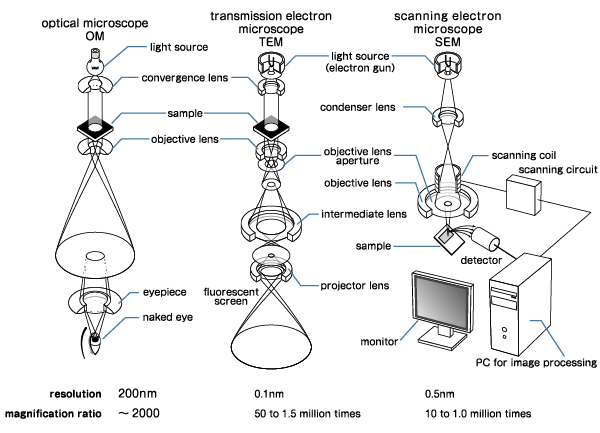Figure: Structure of Electron Microscope

Of these, the lenses correspond to convergence lenses, objective lenses, intermediate lenses, eyepieces, and projector lenses. Compared to an optical microscope, the structure of the lens itself is different even if the same name "lens" is used because an electron beam is used as the light source.

To converge the electron beam, we do not use a material such as glass, but use an electromagnetic action such as a magnet. Therefore, "electronic lenses" such as "electrostatic lenses" and "electromagnetic lenses" are used. There are various types of electronic lenses, but what they have in common is that they utilize electromagnetic action.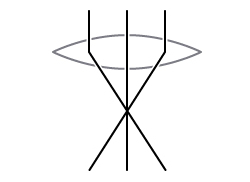optical lenselectronic-lens

Figure: Comparison of Optical Lens and Electronic Lens

We introduce some examples of electronic lenses. An "electrostatic lens (electric field lens)" uses an electric field, especially an electrostatic field, to converge electrons. Basically, the electron beam is converged by arranging the central electrode with a negative charge and the external electrodes with a positive charge above and below the central electrode.

To explain in a little more detail, electrons make motion with constant acceleration in the electric field in the direction perpendicular to the equipotential plane. For example, between parallel electrodes, the equipotential plane is also parallel to the electrodes, so the electrons receive a force that moves between the electrodes in the shortest distance. Therefore, as shown in the figure below, the shape of the equipotential plane is controlled by devising the shape of the external electrode so that the electron can take the desired orbit.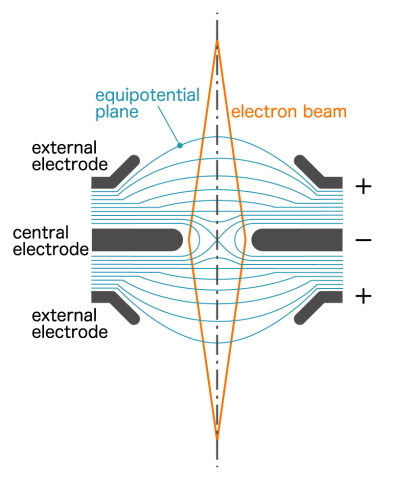Figure: Schematic Diagram of Electrostatic Lens

The figure is a cross section and the actual shape should be axisymmetric (rotational symmetry). This type of lens has the advantage of operating even if the power supply voltage is somewhat unstable. However, there are problems such as large aberrations and the inability to shorten the focal length for high-energy electrons, so we have replaced the "electromagnetic lens" introduced below except for the part that accelerates and decelerates the electrons.

On the other hand, the "electromagnetic lens (magnetic field lens)" uses a magnetic field instead of an equipotential plane. According to Fleming's law, when an electron is incident on an existing magnetic field, a force is applied in the direction perpendicular to the incident direction and the magnetic field. The Lorentz force bends the path of electrons.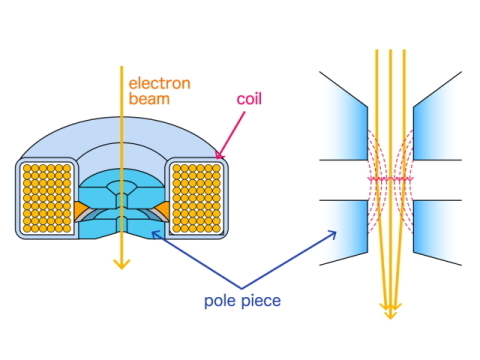Figure: Schematic Diagram of an Electromagnetic Lens

Basically, it is an electromagnet that uses a coil. But since the magnetic field strength is weak (the density of magnetic field lines is low), surround a coil with a material such as iron and create a magnetic pole that protrudes toward the center of the hole. This protruding magnetic pole is called a "pole piece", and the electron beam passes through the area surrounded by this pole piece.

At this time, the electrons passing through the center of the hole are not affected because they are traveling in the same direction as the direction of the magnetic field. But the electrons passing off the center of the hole are affected by the magnetic field and are gathered in the center while drawing a spiral orbit. This is the principle of focusing with an electromagnetic lens.

Since the degree of focusing is affected by the strength of the magnetic field, it is essential that the power supply voltage is stable.

Electromagnetic lenses are less affected by aberrations than electrostatic lenses, but they are still larger than optical lenses. Therefore, when using it, it is necessary to use only the electrons that have passed through the center of the lens as much as possible. The "objective lens diaphragm" placed under the lens plays this role. This cuts electrons from the periphery of the lens, which has large aberrations.

### Positional Relationship between Sample and Lens

Then, what is the positional relationship between the sample and the electronic lens? There are two main sample positions. One is the "out-lens method" in which the electron beam is placed after passing through the objective lens (in the case of SEM) or before (in the case of TEM). The other one is the "in-lens method" in which the electron beam is placed inside the objective lens (in the case of SEM only).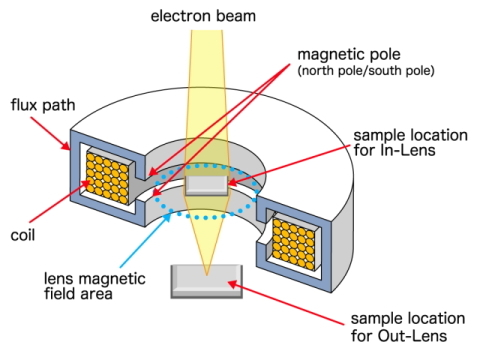Figure: Out-lens Method and In-lens Method

The higher resolution is the in-lens method, which shortens the focal length, but it cannot be used if the sample is affected by a magnetic field. In that case, the out-lens method, which is less affected by the magnetic field, will be used.

In addition, the in-lens method can only target samples of a size that can be placed inside the lens. On the other hand, with the out-lens method, it is possible to target samples that are larger than the in-lens method.

Therefore, it is necessary to use the out-lens method when the sample is large or affected by the magnetic field. And you use the in-lens method when the sample is small and not affected by the magnetic field. However, a "semi-in lens (snorkel) method" objective lens was developed to eliminate this drawback of the in-lens as much as possible.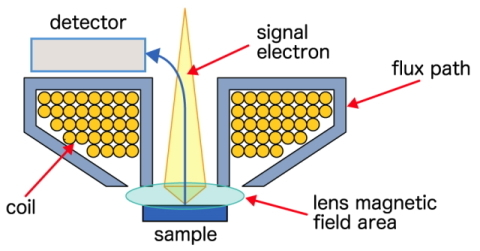Figure: Semi-in-lens Method

Since the sample is placed under the objective lens, it looks like an out-lens type, but the focal length can be shortened by devising the shape of the pole piece, so it is possible to obtain a resolution close to that of the in-lens method. The semi-in-lens method enables high-resolution observation of even large samples.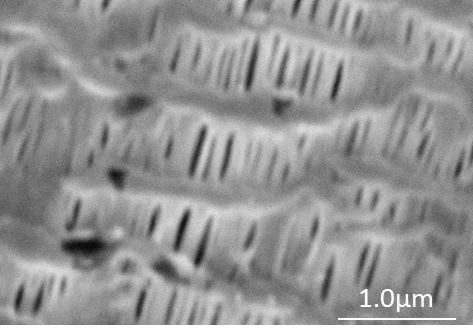(a) Out-lens Method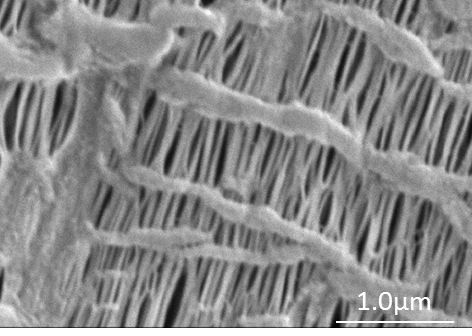(b) Semi-in-lens Method

Figure: Image Comparison between Out-lens Method and Semi-in-lens Method

In addition, in the case of the out-lens method, there is a method of moving the detector closer to the sample for increasing the resolution. In this case, the out lens is an obstacle to inserting the detector. Therefore, it is necessary to increase the focal length, and the advantage of bringing the detector closer is lost.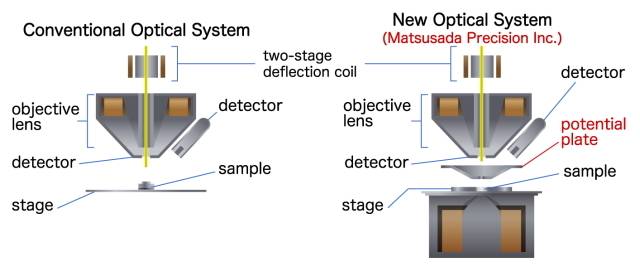Figure: Optical system with bottom lens

This shortcoming is not inevitable. Our "Precision SEM3500 and Precision SEM5600" solves this problem. The optical system of our SEM is as shown in the above figure. In addition to the out lens (top lens), the objective lens (bottom lens, which looks like an inverted semi-in lens) is also placed on the lower side. With this bottom lens, you can improve the resolution by moving the detector closer, and you can use it with a short focal length because the lens is under the sample. With the bottom lens, it is possible to increase the resolution.